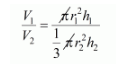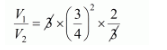# The radii of the base of a cylinder and a cone are`
Question:

The radii of the base of a cylinder and a cone are in the ratio 3 : 4 and their heights are in the ratio 2 : 3. What is the ratio of their volumes?

Solution:

Let r1 and r2 be the radii of the base of a cylinder and a cone.

The volume of cylinder $V_{1}=\pi r_{1}^{2} h_{1} \ldots \ldots . .(i)$

The volume of cone $V_{2}=\frac{1}{3} \pi r_{2}^{2} h_{2} \ldots \ldots$. (ii)

Dividing (i) by (ii), the, we get$\frac{=3 \times\left(\frac{r_{1}}{r_{2}}\right)^{2} \times\left(\frac{h_{1}}{h_{2}}\right)}{\left(\frac{r_{1}}{r_{2}}=\frac{3}{4}, \frac{h_{1}}{h_{2}}=\frac{2}{3}, \text { given }\right)}$$\frac{V_{1}}{V_{2}}=\frac{9}{8}$

$V_{1}: V_{2}=9: 8$